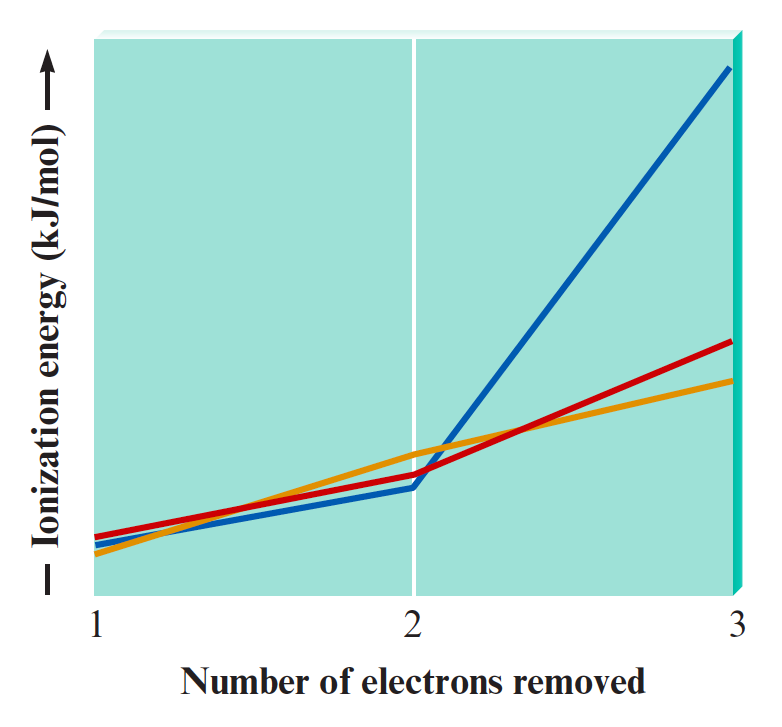# Problem: The following graph plots the first, second, and third ionization energies for Mg, Al, and Si.Without referencing the text, which plot corresponds to which element? In one of the plots, there is a huge jump in energy between I2 and I3, unlike in the other two plots. Explain this phenomenon.

🤓 Based on our data, we think this question is relevant for Professor Holme's class at ISU.

###### FREE Expert Solution

Ionization energy (I. E.) is the energy required to remove an electron from a gaseous atom or ion.

First I.E. corresponds to removing thfirst valence electron from a neutral atom

Second I.E. refers to removing a second electron

Third I.E. refers to removing a third electron.###### Problem Details

The following graph plots the first, second, and third ionization energies for Mg, Al, and Si.Without referencing the text, which plot corresponds to which element? In one of the plots, there is a huge jump in energy between I2 and I3, unlike in the other two plots. Explain this phenomenon.